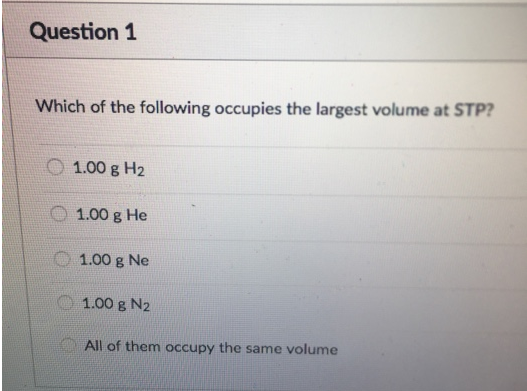Chemistry Practice Problems Chemistry Gas Laws Practice Problems Solution: Which of the following occupies the largest volume...

🤓 Based on our data, we think this question is relevant for Professor Nagavelli's class at HCC.

# Solution: Which of the following occupies the largest volume at STP?a. 1.00 g H2b. 1.00 g Hec. 1.00 g Ned. 1.00 g N2e. All of them occupy the same volume

###### Problem

Which of the following occupies the largest volume at STP?

a. 1.00 g H2

b. 1.00 g He

c. 1.00 g Ne

d. 1.00 g N2

e. All of them occupy the same volumeView Complete Written Solution

Chemistry Gas Laws

Chemistry Gas Laws

#### Q. A fixed quantity of gas at 24°C exhibits a pressure of 736 torr and occupies a volume of 5.46 L. (See the table of physical data.) (a) Calculate the v...

Solved • Wed Jul 04 2018 14:40:55 GMT-0400 (EDT)

Chemistry Gas Laws

#### Q. A fixed quantity of gas at 28°C exhibits a pressure of 737 torr and occupies a volume of 5.50 L. (See the table of physical data.) (a) Calculate the v...

Solved • Wed Jul 04 2018 13:45:30 GMT-0400 (EDT)

Chemistry Gas Laws

#### Q. When the volume of a gas is changed from _________ L to 4.50 L, the temperature will change from 38.1°C to 15.0°C.

Solved • Tue Jul 03 2018 11:54:25 GMT-0400 (EDT)

Chemistry Gas Laws

#### Q. A sample of an ideal gas has a volume of 2.26 L at 288 K and 1.11 atm. Calculate the pressure when the volume is 1.04 L and the temperature is 299 K.

Solved • Mon Jul 02 2018 15:29:45 GMT-0400 (EDT)